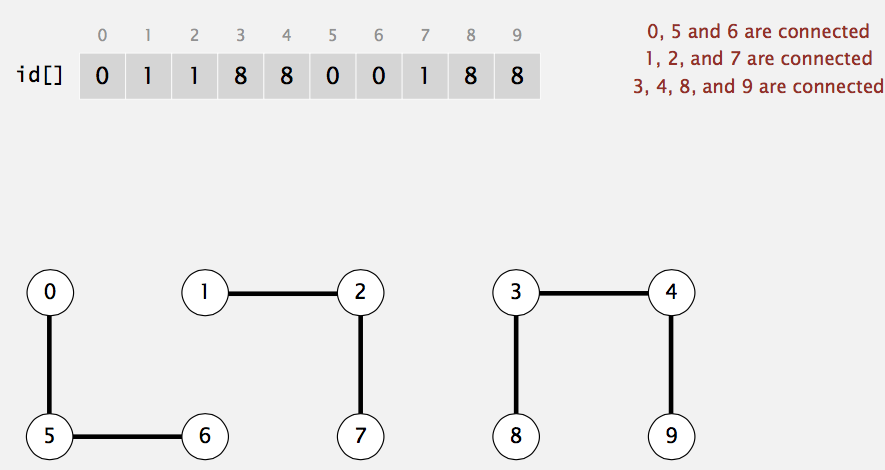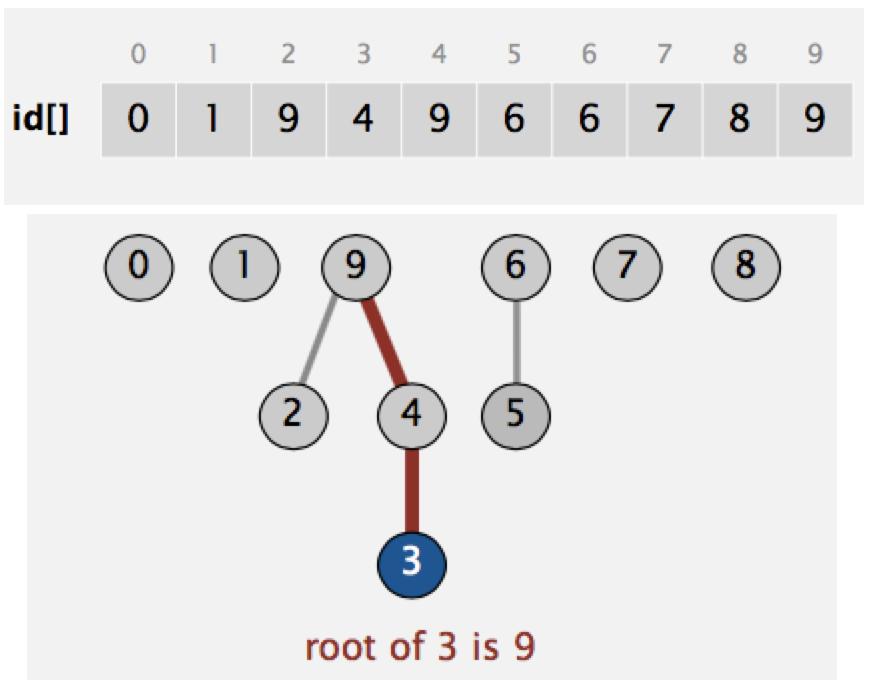# Intro to Union-Find Data Structure

## Jul 13, 2015 · 1005 words · 5 minutes read algorithm course

This post introduces a simple data structure called Union Find. If you wonder how social app may learn whether people A and B may know each other through some common friends, please read on :)

This is my summary of Princeton open course Algorithm, Part I, first week content.

## Problem: Dynamic Connectivity

Given a set of N objects, we want to:

1. Union command: connect two objects.
2. Find/connected query: is there a path connecting the two objects?

As shown in the following GIF, there are N=10 objects, we do 5 unions and then check object and object are not connected, object and object are connected. After 4 more unions, object and object are connected.

Below is another example. Obviously, it’s hard for human to figure out whether point p and q are connected or not, we need computer to do that.

### Applications

As you can guess, there are many applications on this:

• Friends in a social network
• Pixels in a digital photo
• Computers in a network
• Transistors in a computer chip
• Metallic sites in a coposite system

### Model Building

When programming, it’s convenient to name objects 0 to N-1. Because:

• Use integers as array index
• Suppress details not relevant to union-find. (can use symbol table to translate from site names to integers)

We assume connectivity is an equivalence relation, which is reflexive, symmetric, and transitive.

Then we define Connected Components: Maximal set of objects that are mutually connected. Example below.

### Union-find Data Type (API)

Our goal is: Design efficient data structure for union-find.

• Number of objects N can be huge
• Number of operations M can be huge
• Find queries and union commands may be intermixed.

Let’s figure out how to implement Union-find next.

## Quick Find

How would you represent the data structure?

One way we can do is:

• use Integer array id[] of lenght N
• p and q are connected iff (if and only if) they have the same id
• when merge components containning p and q, change all entries whose id equals id[p] to id[q]Quick Find Example

### Java Implementation

``````public class QuickFindUF {
private int[] id; // id[i] is component id for object i
public QuickFindUF(int N) {
id = new int[N];
for (int i = 0; i < N; ++i)
id[i] = i;
}

public boolean connected(int p, int q) {
return id[p] == id[q];
}

public void union(int p, int q) {
int pid = id[p];
int qid = id[q];
for (int i = 0; i < id.length; ++i)
if (id[i] == pid) id[i] = qid;
}
}
``````

As you can see, quick-find is simple but slow. Even though number of arrray accesses for find/connected is O(1), but union is O(N), which leads to O(N^2) array accesses to process a sequence of N union commands on N objects.

## Quick Union

Another way to represent the data structure is:

• integer array id[] of length N, where id[i] is parent of i
• root of i is id[id[…id[i]…]] (keep going until it doesn’t change)
• for find: check if p and q have same root
• for union: merge components containing p and q, set the id of p’s root to the id of q’s rootQuick Union Example

### Java Implementation

``````public class QuickUnionUF {
private int[] id; // id[i] is parent of i

public QuickUnionUF(int N) {
id = new int[N];
for (int i = 0; i < N; ++i)
id[i] = 1;
}

private int root(int i) {
while (i != id[i])
i = id[i];
return i;
}

public boolean connected(int p, int q) {
return root(p) == root(q);
}

public void union(int p, int q) {
int i = root(p);
int j = root(q);
id[i] = j;
}
}
``````

Becuase trees can get tall (imagine union objects in sequence of 1,2,…N-1), the union and find operation are O(N). Still not good.

## Improvements

### Weighting

Is there a way to avoid tall trees? Sure there is – weighted quick-union:

• modify quick-union to avoid tall trees.
• keep track of size of each tree (number of objects).
• balance by linking root of smaller tree to root of larger tree.

To implement that, we need to define an extra array sz[i] to count number of objects in the tree rooted at i. When do union operation, we link root of smaller tree to root of larger tree, and update the sz[] array.

``````int i = root(p);
int j = root(q);
if (i == j) return;
if (sz[i] < sz[j]) { id[i] = j; sz[j] += sz[i]; }
else               { id[j] = i; sz[i] += sz[j]; }
``````

This time, both find and union take O(lgN), because root function takes O(lgN). The root takes O(lgN) because the depth of any node x is at most lgN. Why? When a tree T1 containing node x need to union with another tree T2, the depth of x only increase 1 if `|T2| >= |T1|`, and after union, the size of tree containing x at least doubles. How many times can size of tree containing x double? lgN times.

The weighting gives us acceptable performance (O(lgN)), but we can improve further very easily.

### Path Compression

To further improve weighted quick-union, we want to descrese the depth of the tree. The idea is: when we perform root operation, why not just point every node you examed to the root?

Two implementations:

• Two-pass: add second loop to root() to set the id[] of each examined node to the root.
• Simpler one-pass variant: make every other node in path point to its grandparent (thereby halving path length).
``````private int root(int i) {
while (i != id[i]) {
id[i] = id[id[i]]; // only one extra line of code
i = id[i];
}
return i;
}
``````

## Summary

To solve the Dynamic Connectivity problem, we introduce union-find data structure. The quick-find implementation support fast (O(1)) find operation, but very slow union. The quick-union implementation is slow on both find and union, but we can use weighting and path compression to achieve O(lgN) cost on both find and union.

In next post, let’s use union-find data structure to solve some problems.